# Using PCA to identify correlated stocks in Python

## Overview

Principal component analysis is a well known technique typically used on high dimensional datasets, to represent variablity in a reduced number of characteristic dimensions, known as the principal components.

In this post, I will show how PCA can be used in reverse to quantitatively identify correlated time series. We will compare this with a more visually appealing correlation heatmap to validate the approach.

### The plan:

1. Clean and prepare the data
2. Check for stationarity
3. Generate qualitative correlation matrix
4. Perform PCA
5. Generate loadings plots
6. Quantitatively identify and rank strongest correlated stocks

## Using PCA in reverse

This approach is inspired by this paper, which shows that the often overlooked ‘smaller’ principal components representing a smaller proportion of the data variance may actually hold useful insights.

The authors suggest that the principal components may be broadly divided into three classes:

• The top few components which represent global variation within the dataset.
• A set of components representing the syncronised variation between certain members of the dataset.
• Components representing random fluctuations within the dataset.

Now, the second class of components is interesting when we want to look for correlations between certain members of the dataset. For example, considering which stock prices or indicies are correlated with each other over time. This is the application which we will use the technique.

## The raw data

First, some data. Three real sets of data were used, specifically. Daily closing prices for the past 10 years of:

• A selection of stocks representing companies in different industries and geographies.
• A selection of industry indexes.
• A selection of geography indexes.

These files are in CSV format. They are imported as data frames, and then transposed to ensure that the shape is: dates (rows) x stock or index name (columns).

## Cleaning and pre-processing

Pandas dataframes have great support for manipulating date-time data types. However the dates for our data are in the form “X20010103”, this date is 03.01.2001. So a dateconv function was defined to parse the dates into the correct type.

This was then applied to the three data frames, representing the daily indexes of countries, sectors and stocks repsectively.

trimmer = lambda x: x[1:]

def dateconv(dataframe,func):
dataframe = dataframe.reset_index() #reindex so we can manipultate the date field as a column
dataframe['index'] = dataframe['index'].apply(func) #remove the trailing X
dataframe['index'] = pd.to_datetime(dataframe['index']) #convert the index col to datetime type
dataframe = dataframe.set_index('index') #restore the index column as the actual dataframe index
dataframe = dataframe[1:]
return dataframe

countriesT = dateconv(countriesT,trimmer)
sectorsT = dateconv(sectorsT,trimmer)
stocksT = dateconv(stocksT,trimmer)


This is done because the date ranges of the three tables are different, and there is missing data. For example the price for a particular day may be available for the sector and country index, but not for the stock index. Ensuring pandas interprets these rows as dates will make it easier to join the tables later.

### Log returns

As the stocks data are actually market caps and the countries and sector data are indicies. We need a way to compare these as relative rather than absolute values. The market cap data is also unlikely to be stationary - and so the trends would skew our analysis.

So, instead, we can calculate the log return at time t, $R_{t}$ defined as:

### Merging the data

Now, we join together stock, country and sector data. As not all the stocks have records over the duration of the sector and region indicies, we need to only consider the period covered by the stocks. To do this, create a left join on the tables: stocks<-sectors<-countries.

So the dimensions of the three tables, and the subsequent combined table is as follows:

Dims of stocks: (1520, 11) # 11 stocks
Dims of sectors: (4213, 54) # 54 sector indicies
Dims of countries: (4213, 25) # 25 country indicies
Dims of combined data table: (1520, 90)


Now, finally we can plot the log returns of the combined data over the time range where the data is complete: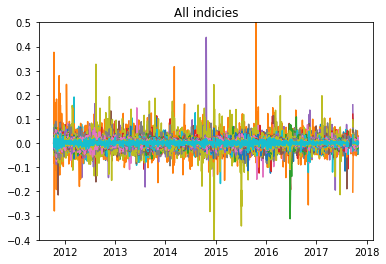### Checking for stationary data

It is important to check that our returns data does not contain any trends or seasonal effects. There are a number of ways we can check for this

1. ADF test

The null hypothesis of the Augmented Dickey-Fuller test, states that the time series can be represented by a “unit root”, (i.e. it has some time dependent structure). Rejecting this null hypothesis means that the time series is stationary. If the ADF test statistic is < -4 then we can reject the null hypothesis - i.e. we have a stationary time series.

The adfuller method can be used from the statsmodels library, and run on one of the columns of the data, (where 1 column represents the log returns of a stock or index over the time period). In this case we obtain a value of -21, indicating we can reject the null hypothysis.

2. Inspection of the distribution

We can also plot the distribution of the returns for a selected series. If this distribution is approximately Gaussian then the data is likely to be stationary. Below, three randomly selected returns series are plotted - the results look fairly Gaussian.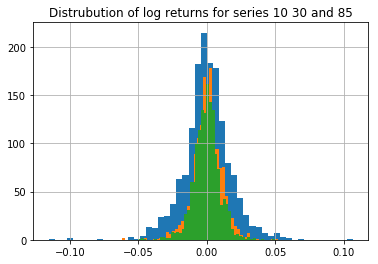### Calculating the correlation matrix

We can now calculate the covariance and correlation matrix for the combined dataset. We will then use this correlation matrix for the PCA. (The correlation matrix is essentially the normalised covariance matrix)

Before doing this, the data is standardised and centered, by subtracting the mean and dividing by the standard deviation.

#manually calculate correlation coefficents - normalise by stdev.
combo = combo.dropna()
m = combo.mean(axis=0)
s = combo.std(ddof=1, axis=0)

# normalised time-series as an input for PCA
combo_pca = (combo - m)/s

c = np.cov(combo_pca.values.T)     # covariance matrix
co = np.corrcoef(combo_pca.values.T) #correlation matrix


Using Plotly, we can then plot this correlation matrix as an interactive heatmap:

We can see some correlations between stocks and sectors from this plot when we zoom in and inspect the values. Some noticable hotspots from first glance:

• stock 7332687^ and the Nordic region (0.77)
• stock 6900212^ and the Japan Homebuilding market (0.66)
• stock 2288406^ and Oil & Gas (0.65)

### Performing PCA

Perfomring PCA involves calculating the eigenvectors and eigenvalues of the covariance matrix. The eigenvectors (principal components) determine the directions of the new feature space, and the eigenvalues determine their magnitude, (i.e. the eigenvalues explain the variance of the data along the new feature axes.)

First, we decompose the covariance matrix into the corresponding eignvalues and eigenvectors and plot these as a heatmap.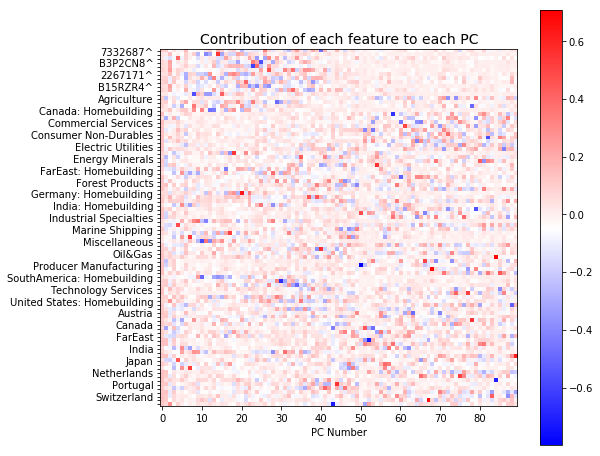This plot shows the contribution of each index or stock to each principal component. There are 90 components all together. We can see that the early components (0-40) mainly describe the variation across all the stocks (red spots in top left corner). It also appears that the variation represented by the later components is more distributed.

The total variability in the system is now represented by the 90 components, (as opposed to the 1520 dimensions, representing the time steps, in the original dataset). The eigenvalues can be used to describe how much variance is explained by each component, (i.e. how the varaiance is distributed across our PC’s).

Below we plot this distribution:

As we can see, most of the variance is concentrated in the top 1-3 components. These components capture “market wide” effects that impact all members of the dataset.

Following the approach described in the paper by Yang and Rea, we will now inpsect the last few components to try and identify correlated pairs of the dataset

### Developing the loadings plot

As mentioned earlier, the eigenvalues represent the scale or magnitude of the variance, while the eigenvectors represent the direction. The “loadings” is essentially the combination of the direction and magnitude. The loading can be calculated by “loading” the eigenvector coefficient with the square root of the amount of variance:

We can plot these loadings together to better interpret the direction and magnitude of the correlation. The loadings for any pair of principal components can be considered, this is shown for components 86 and 87 below:

The loadings plot shows the relationships between correlated stocks and indicies in opposite quadrants. Indicies plotted in quadrant 1 are correlated with stocks or indicies in the diagonally opposite quadrant (3 in this case). The length of the line then indicates the strength of this relationship.

For example, stock 6900212^ correlates with the Japan homebuilding market, as they exist in opposite quadrants, (2 and 4 respectively). This is consistent with the bright spots shown in the original correlation matrix.

### Using the loadings plots to identify and rank correlated stocks

We can use the loadings plot to quantify and rank the stocks in terms of the influence of the sectors or countries.

To do this, we categorise each of the 90 points on the loading plot into one of the four quadrants. Then, we look for pairs of points in opposite quadrants, (for example quadrant 1 vs 3, and quadrant 2 vs 4). Then, if one of these pairs of points represents a stock, we go back to the original dataset and cross plot the log returns of that stock and the associated market/sector index.

Using the cross plot, the $R^2$ value is calculated and a linear line of best fit added using the linregress function from the stats library. A cutoff $R^2$ value of 0.6 is then used to determine if the relationship is significant.

Cross plots for three of the most strongly correlated stocks identified from the loading plot, are shown below: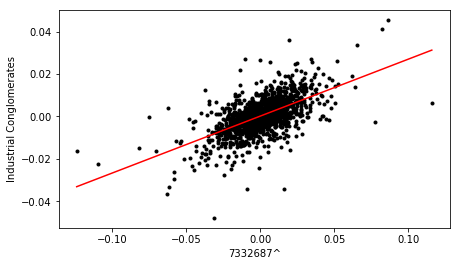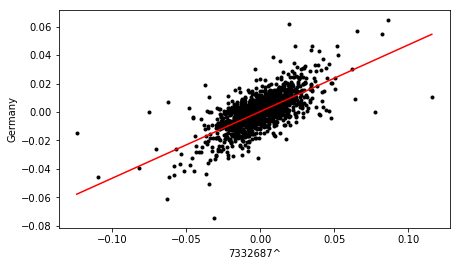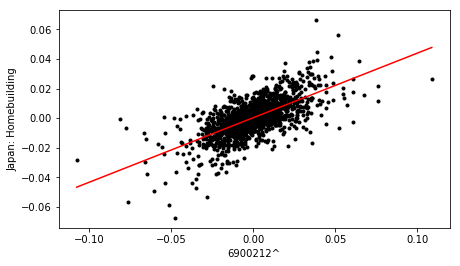Finally, the dataframe containing correlation metrics for all pairs is sorted in terms descending order of $R^2$ value, to yield a ranked list of stocks, in terms of sector and country influence.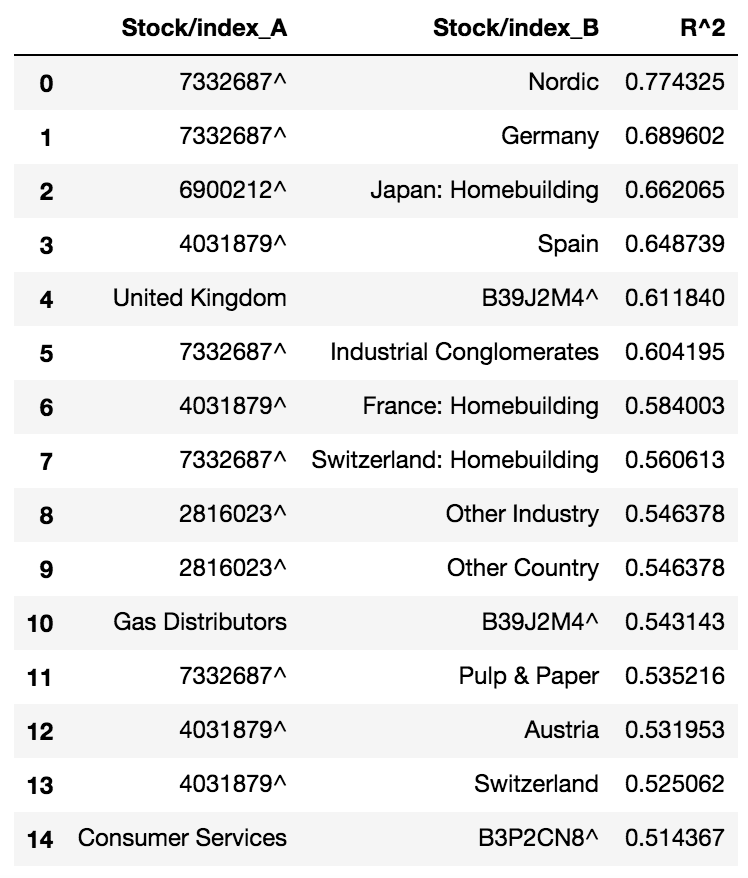The top correlations listed in the above table are consistent with the results of the correlation heatmap produced earlier.

This analysis of the loadings plot, derived from the analysis of the last few principal components, provides a more quantitative method of ranking correlated stocks, without having to inspect each time series manually, or rely on a qualitative heatmap of overall correlations. It would be cool to apply this analysis in a ‘sliding window’ approach to evaluate correlations within different time horizons.

Hope you found it interesting!

You can find the full code for this project here

# How to run Jupyter notebooks on AWS with a reverse proxy

I needed to host a Python Jupyter Notebook remotely and then use this at work to do some data analysis with python. The problem was that the company firewall filtering was blocking ports stopping me accesssing the “bare” ubuntu instance running Jupyter.

To get around this, i setup an Nginx reverse proxy in front of the Jupyter server. This allowed regular HTTP access requests from outside clients on port 80, to be directed to the jupyter server. Maybe this setup will be useful to others who want a hosted Jupyter server.

## The setup

The diagram below shows the setup. Basically, an Nginx reverse proxy server will proxy requests from the client back to our jupyter web app running on an AWS EC2.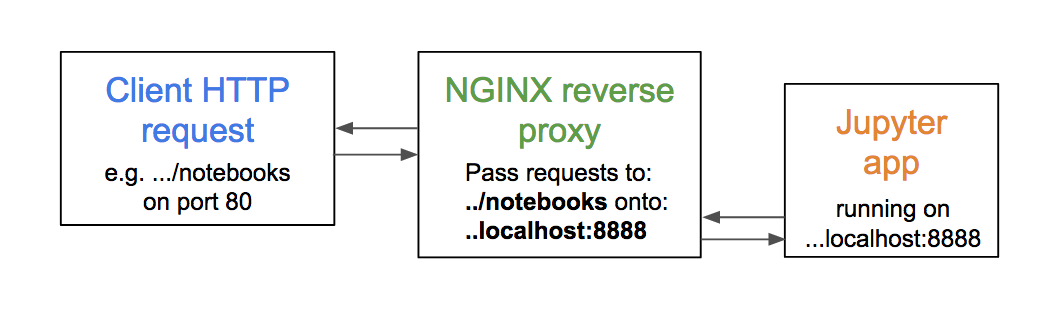### 1. Launch an Ubuntu EC2 instance

First up, sign into the AWS console, head over to the EC2 section and hit launch instance. Select the Ubuntu Server 16.04 LTS (HVM) SSD AMI.Follow through the steps to launch the instance. I just chose the t2.micro tier with 1 CPU for running fairly light python tasks and it costs around $10 per month. (If you’re a student its worth checking out if you can claim free AWS credits :). ### 2. Configure the Instance Setup the inbound security rules as follows. You can leave the outbound rules as the default.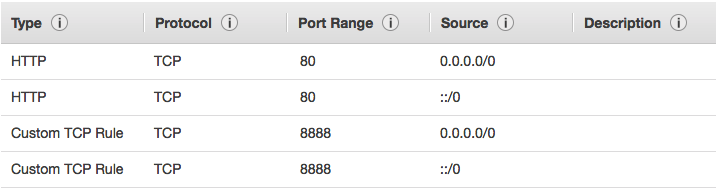Next, open up a terminal and SSH into the instance. We will now install Python and Jupyter using the Anaconda distribution. To do this, go here, right click on the green download link and copy the URL.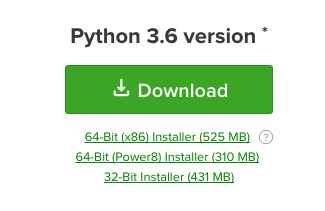Then, run the following commands to download and install python on your instance using anaconda: $ wget http://repo.continuum.io/archive/Anaconda3-4.1.1-Linux-x86_64.sh # or your pasted URL copied above
$bash Anaconda3-4.1.1-Linux-x86_64.sh  Answer yes when it asks to update your PATH environment variable. Anaconda will also install Jupyter and some other useful libraries such as numpy. To check the installation was performed, run the following: $ which python3


and you should get something like /home/ubuntu/anaconda3/bin/python3

### 3. Configure Jupyter

Next, we need to setup a password for the accessing notebook. This will be useful to restrict access to the notebook when we have the reverse proxy setup.

To do this, first enter the ipython enviroment using:

$ipython  Once in the enviroment, enter the following: from IPython.lib import passwd passwd()  This will ask you to enter a password. Enter anything you like, and once verified, the hash of your chosen password is given. Copy this hash and paste it somewhere for later. Next, we need setup the jupyter configuration file. The following command will generate a default configuration file which we can then update: $ jupyter notebook --generate-config


By default, this will generate the configuration file jupyter_notebook_config.py which is located under the .jupyter folder in the home directory. Using nano or vim, open and edit this config file as follows.

c.NotebookApp.base_url = '/notebook/'
c.NotebookApp.port = 8888
c.NotebookApp.trust_xheaders = True
c.NotebookApp.port_retries = 50
c.NotebookApp.password = paste your hashed value from earlier


The NotebookApp.base_url and NotebookApp.port will need to match with the proxy setup in the next section. In this case, we will configure nginx to proxy incoming requests to port 8888 where the jupyter notebook app will be running.

### 4. Setup Nginx

Nginx is a popular HTTP and reverse proxy server. We will setup Nginx to act as a web server, listening for HTTP requests on port 80. If these requests are made to the correct URL, Nginx will direct these requests to port 8888 where the jupyter notebook will be running.

First, install Nginx:

$sudo apt-get update$ sudo apt-get install nginx


Once this has been installed, navigate to the Nginx configuration file located at /etc/nginx/site-enabled/default. Update the server block to the following:

server {
location /notebook {
proxy_pass http://localhost:8888;
proxy_set_header X-Forwarded-For $proxy_add_x_forwarded_for; proxy_set_header X-Real-IP$remote_addr;
proxy_set_header Host $http_host; proxy_http_version 1.1; proxy_redirect off; proxy_buffering off; proxy_set_header Upgrade$http_upgrade;
proxy_set_header Connection "upgrade";
proxy_read_timeout 86400;
} ...



With this setup, when a request to /notebook is made, Nginx will proxy this to localhost:8888, where the jupyter notebook is running.

Once you have made these updates, you can restart Nginx using the following:

$sudo service nginx restart  Now, go to your AWS console, select your running instance, and copy the Public DNS as listed in the bottom right hand corner of the Description tab. Paste this address in a new tab, and append this with /notebook. Enter the password which you set earlier, and you should then gain access to your Jupyter notebook server! ### 5. Automatic restart of the server While working on your notebooks you can save your work using the top left hand menu. When you shutdown and subsequently restart your instance, these changes will be preseved. However when you shutdown and restart the instance Jupyter will not automatically restart. So, to save you having to SSH into your instance and start this manually each time, we can use cron to have the server and Jupyter start on every @reboot event. To do this, run the following command to create a crontab for your ubuntu instance: $ crontab -e


When asked, select nano as your editor. In the crontab, using Nano, edit the file to add the following:

@reboot nohup jupyter notebook
@reboot cd /home/ubuntu; source ~/.bashrc;  /home/ubuntu/anaconda3/bin/jupyter



The part of the last line /home/ubuntu/anaconda3/bin/jupyter  should be the output of which python3 from step 2. This is required so that cron sources the correct path for the python installation.

Now, if you shutdown and restart your instance, your Jupyter notebook server should have automatically restarted and be ready for you when you hit the \notebook URL.

Hope thats helpful!

# Buckley Leverett analysis in Python

I thought it would be interesting to write some functions to perform a Buckley-Leverett analysis using python and matplotlib.

### The theory

The Buckley-Leverett partial differential equation is:

As $S_w(x,t)$ we can write the total derivative as:

and, given that the fluid front is of constant saturation in the analysis, we can say:

Substituting this into the Buckley-Leverett equation, gives:

Integration of both sides over time $t=0$ to $t=t$:

gives an expression for the position of the fluid front $x_f$ at time $t$:

As we know that the fractional flow in the simple case of horizontal flow can be calculated as:

we can plot this, along with it’s derivative $\frac{df_w}{dS_w}$ calculated numerically: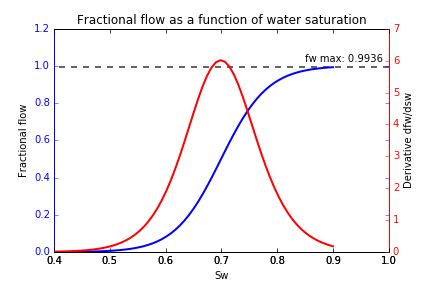To determine the water saturation at the shock front, we can construct chords starting at the connate water saturation, working our way up the fractional flow curve until we find the maximum gradient - this point gives us the shock saturation. In this case, $S_w = 0.81$: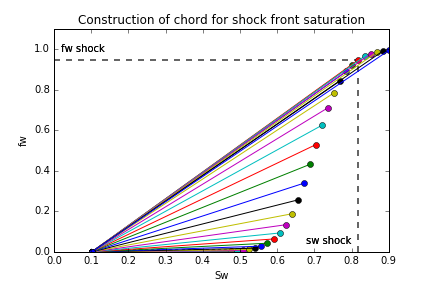Then we can plot the saturation as a function of the position $x_f$.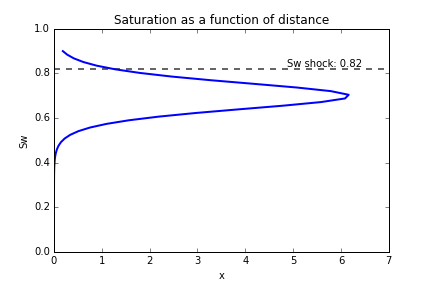The curve suggests there exists two saturation solutions at each distance step, which is not physically possible. The shock front saturation is a discontinuity, and this can be used to modify the plot above to yield a profile of the saturation behind and in front of the saturation front.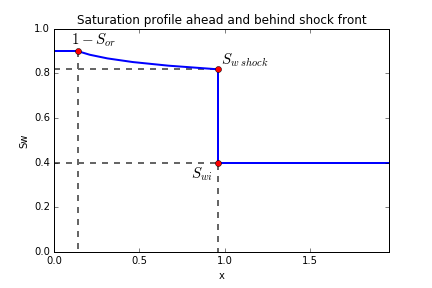• $S_{w i}$ represents the initial water saturation, where the shock front has not yet reached
• $S_{w \ shock}$ represents the water saturation at the shock front
• $1-S_{or}$ represents the water saturation behind the shock front, after the saturation front has swept through, this location is left at the residual oil saturation, $S_{or}$ .

### The code

The Buckley-Leverett class contains the relevant attributes for each instance, including the permeabiliy end points which can be used to generate the relative permeability curves using Corey type relationships.

class BuckleyLev():

def __init__(self):
self.params = {
#non wetting phase viscosity
"viscosity_o": 1.e-3,
#wetting phase viscosity
"viscosity_w": 0.5e-3,
#initial water sat
"initial_sw":0.4,
#irreducable water saturation, Swirr
"residual_w":0.1,
#residual oil saturation, Sor
"residual_o":0.1,
#water rel perm at water curve end point
"krwe":1,
#oil rel perm at oil curve end point
"kroe": 0.9,
#porosity
'poro':0.24,
#water injection rate units m3/hr
"inject_rate":200,
#cross sectional area units m2
"x-area":30
}


Using the these instance attributes we can then add methods to the class for calculating the fractional flow:

def fractional_flow(self,sw):
#returns the fractional flow

return 1./(1.+((self.k_rn(sw)/self.k_rw(sw))*(self.params["viscosity_w"]/self.params["viscosity_o"])))

BuckleyLev.fractional_flow = fractional_flow


and for calculating the derivative numerically a finite difference approximation:

def fractional_flow_deriv(self,sw):
#calculate derivative of fractional flow - dFw/dSw - Vsh

f_deriv = (self.fractional_flow(sw+0.0001) - self.fractional_flow(sw))/0.0001

return f_deriv

BuckleyLev.fractional_flow_deriv = fractional_flow_deriv


The full code is available here

By defining the attirbutes in this way, we can also visualise at the effects of evaluating the position of the shock as it progresses at different time steps, as follows: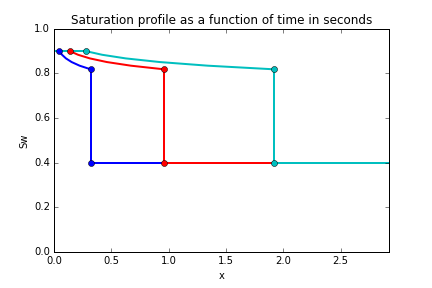Another interesting property is the actual proerties of the fluids involved in the displacement. The mobility ratio is defined as $\frac{k_{ro}}{\mu_o}$. For a higher viscosity oil, the mobility ratio is lower. We can see the effects of this in the below figure - a more viscous oil means the water brekthrough occurs earlier.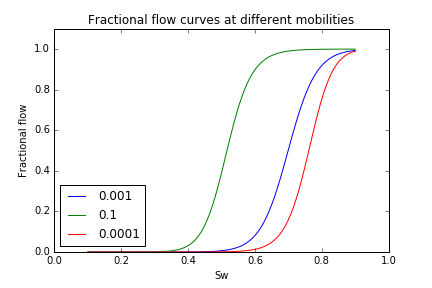# Kidney Stone Calcium Oxalate Crystallisation Modelling

A project from a few years ago, writeup coming soon...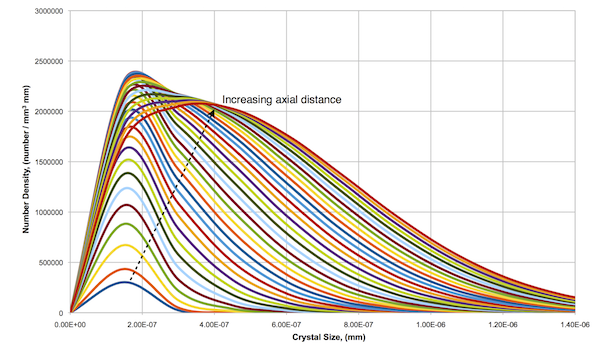# Creativity + Engineering = cool stuff

Hello and welcome to my first blog post. I plan to use this blog as a platform to demonstrate cool projects and ideas that i’ve been working on. Check back soon for the latest on this!

Thanks!